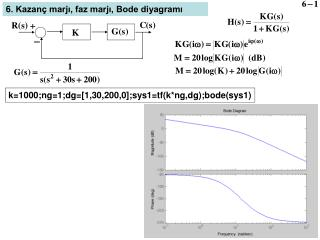Download Presentationk= 1 000;ng=1;dg=[1,30,200,0];sys1=tf(k*ng,dg);bode(sys1)

k= 1 000;ng=1;dg=[1,30,200,0];sys1=tf(k*ng,dg);bode(sys1) - PowerPoint PPT Presentation

6 . Kazanç marjı, faz marjı , Bode diyagramı. k= 1 000;ng=1;dg=[1,30,200,0];sys1=tf(k*ng,dg);bode(sys1). Nyquist diyagramı. Bode diyagramı. [ gm ,pm,w2,w1]=margin(sys1). K=2000: gm=3, pm=32.61, w2=14.14 rad/s, w1=7.49 rad/s. K=2678.6: gm=2.24, pm=23.52 o , w2=14.14 rad/s, w1=9.05 rad/s.I am the owner, or an agent authorized to act on behalf of the owner, of the copyrighted work described.
Download Presentationk= 1 000;ng=1;dg=[1,30,200,0];sys1=tf(k*ng,dg);bode(sys1)

Download Policy: Content on the Website is provided to you AS IS for your information and personal use and may not be sold / licensed / shared on other websites without getting consent from its author.While downloading, if for some reason you are not able to download a presentation, the publisher may have deleted the file from their server.

- - - - - - - - - - - - - - - - - - - - - - - - - - E N D - - - - - - - - - - - - - - - - - - - - - - - - - -
Presentation Transcript
1. 6. Kazanç marjı, faz marjı, Bode diyagramı k=1000;ng=1;dg=[1,30,200,0];sys1=tf(k*ng,dg);bode(sys1)

2. Nyquist diyagramı Bode diyagramı [gm,pm,w2,w1]=margin(sys1) K=2000: gm=3, pm=32.61, w2=14.14 rad/s, w1=7.49 rad/s

3. K=2678.6: gm=2.24, pm=23.52o, w2=14.14 rad/s, w1=9.05 rad/s 9.05 rad/s (1.44 Hz) te 65-23.52=41.48o faz ekle Faz ekle (phase-lead) devresi ωM=9.05: T=0.0498 φM=41.48 : a=4.92

4. Faz azalt (phase-lag) devresi Faz ekle-azalt (phase lead-lag) devresi Faz ekle devresi: PM artar, sönüm artar, aşma azalır, ess değişmez Faz azalt devresi: PM azalır, sönüm azalır, ess (1/a) kadar azalır

5. 0-ωb : Band genişliği (BW) Rezonans Köşe frekansı=a (rad/s)